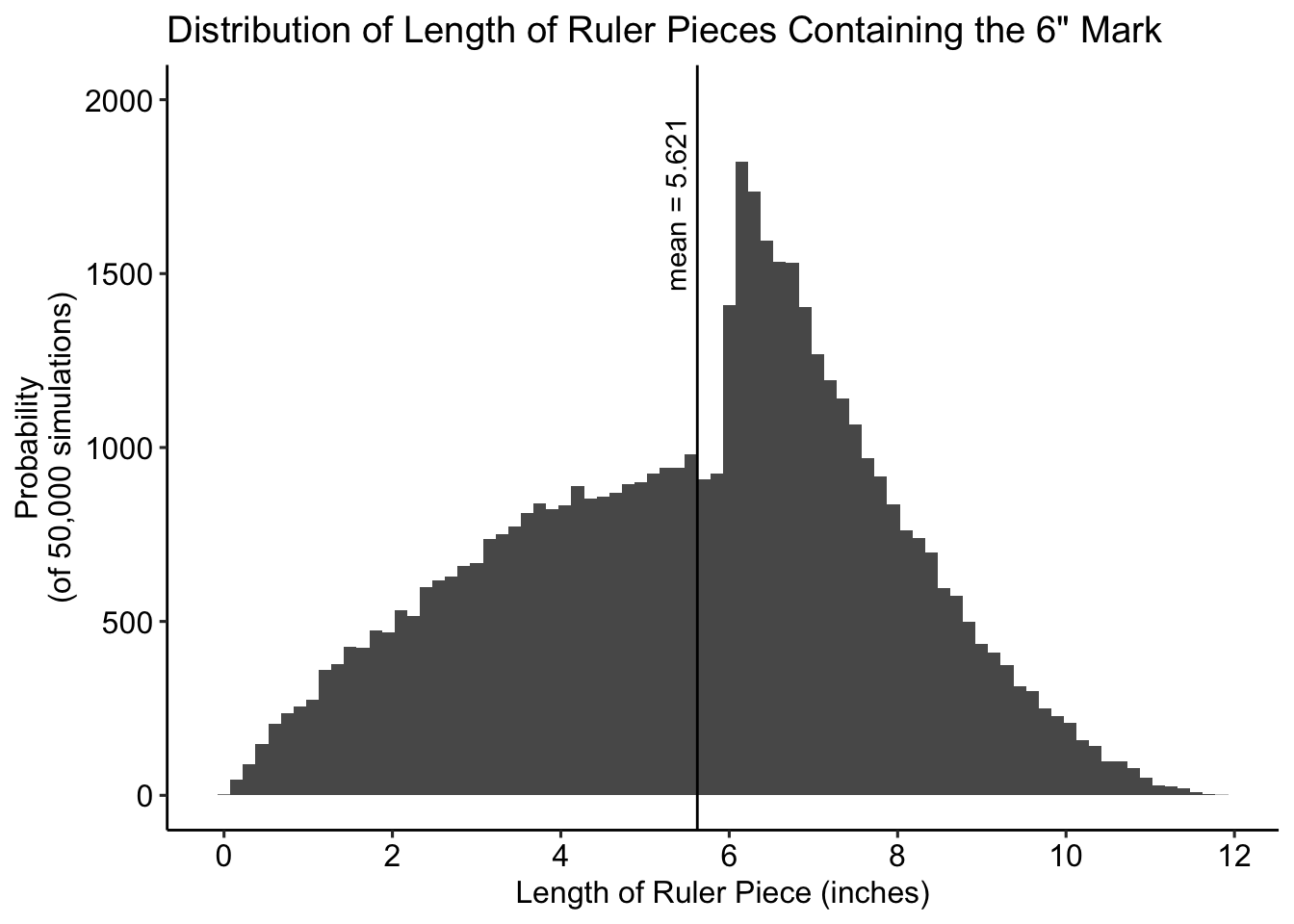### Riddler Classic

#### By Zach Wissner-Gross

From Angela Zhou comes one riddle to rule them all:

The Riddler Manufacturing Company makes all sorts of mathematical tools: compasses, protractors, slide rules — you name it!

Recently, there was an issue with the production of foot-long rulers. It seems that each ruler was accidentally sliced at three random points along the ruler, resulting in four pieces. Looking on the bright side, that means there are now four times as many rulers — they just happen to have different lengths.

On average, how long are the pieces that contain the 6-inch mark?

### My Solution

Math is for nerds, so I’ll just simulate this.

breakRuler <- function (rulerLength = 12, breakNum = 3, mark = 6) {
breaks <- sample(seq(0, rulerLength, by = 0.001), breakNum) # hopefully 0.001 is small enough
breaks <- breaks[order(breaks)]

pieceLengths <- c()
hasMark <- c()

for (pieceNum in 1:(length(breaks) + 1)) {
pieceBreak <- breaks[pieceNum]

if (pieceNum == 1) {
pieceLengths <- pieceBreak
if (pieceBreak >= mark) {
hasMark <- T
} else {
hasMark <- F
}

} else if (pieceNum <= length(breaks)){
pieceLengths <- c(pieceLengths, (pieceBreak - breaks[pieceNum - 1]))
if (pieceBreak >= mark && breaks[pieceNum - 1] < mark) {
hasMark <- c(hasMark, T)
} else {
hasMark <- c(hasMark, F)
}

} else {
pieceLengths <- c(pieceLengths, (rulerLength - breaks[pieceNum - 1]))
if (breaks[pieceNum - 1] < mark) {
hasMark <- c(hasMark, T)
} else {
hasMark <- c(hasMark, F)
}
}
}
return(pieceLengths[hasMark])
}
allLengths <- c()
for (i in 1:50000) {
allLengths <- c(allLengths, breakRuler())
}
print(paste("The mean length of ruler pieces that contain the 6 inch mark is", mean(allLengths)))
##  "The mean length of ruler pieces that contain the 6 inch mark is 5.621061"
print(paste("The median length of ruler pieces that contain the 6 inch mark is", median(allLengths)))
##  "The median length of ruler pieces that contain the 6 inch mark is 6.005"
library(ggplot2)
library(ggpubr)

ggplot(data.frame(len = allLengths)) +
geom_histogram(aes(x = len), binwidth = 0.15) +
geom_vline(xintercept = mean(allLengths)) +
annotate(geom = "text", x = mean(allLengths) - 0.25, y = 1700,
label = paste("mean =", round(mean(allLengths), digits = 3)),
angle = 90) +
ylim(c(0, 2000)) +
ylab("Probability\n(of 50,000 simulations)") +
scale_x_continuous(name = "Length of Ruler Piece (inches)",
breaks = c(seq(0, 12, by = 2))) +
ggtitle("Distribution of Length of Ruler Pieces Containing the 6\" Mark") +
theme_pubr()What are the average lengths of ruler pieces that contain the other marks? Here’s code for an animation:

for (rulerMark in 0:12) {
allLengths <- c()
for (i in 1:50000) {
allLengths <- c(allLengths, breakRuler(mark = rulerMark))
}
print(paste("The mean length of pieces that contain the", rulerMark, "mark is", mean(allLengths)))
ggplot(data.frame(len = allLengths)) +
geom_histogram(aes(x = len), binwidth = 0.15) +
geom_vline(xintercept = mean(allLengths)) +
annotate(geom = "text", x = mean(allLengths) - 0.25, y = 1700,
label = paste("mean =", round(mean(allLengths), digits = 3)),
angle = 90) +
ylim(c(0, 2000)) +
ylab("Probability\n(of 50,000 simulations)") +
scale_x_continuous(name = "Length of Ruler Piece (inches)",
breaks = c(seq(0, 12, by = 2)),
limits = c(0, 12)) +
ggtitle(paste("Length of Ruler Piece Containing ", rulerMark, "\" Mark", sep = "")) +
theme_pubr()
ggsave(paste("animMarks/hist",
formatC(rulerMark, digits = 1, format = "d", flag = "0"),
".png",
sep = ""))
}

What happens if we iterate on how many breaks there are? Here’s code for an animation:

for (rulerBreaks in 1:12) {
allLengths <- c()
for (i in 1:50000) {
allLengths <- c(allLengths, breakRuler(breakNum = rulerBreaks))
}
print(paste("The mean length of pieces that contain the 6\" mark with ",
rulerBreaks, " breaks is ", mean(allLengths)))
ggplot(data.frame(len = allLengths)) +
geom_histogram(aes(x = len), binwidth = 0.15) +
geom_vline(xintercept = mean(allLengths)) +
annotate(geom = "text", x = mean(allLengths) + 0.25, y = 2700,
label = paste("mean =", round(mean(allLengths), digits = 3)),
angle = 90) +
ylim(c(0, 3000)) +
ylab("Probability\n(of 50,000 simulations)") +
scale_x_continuous(name = "Length of Ruler Piece (inches)",
breaks = c(seq(0, 12, by = 2)),
limits = c(0, 12)) +
ggtitle(paste("Length of Ruler Piece Containing 6\" Mark with", rulerBreaks, "Break(s)")) +
theme_pubr()
ggsave(paste("animBreaks/hist",
formatC(rulerBreaks, digits = 1, format = "d", flag = "0"),
".png",
sep = ""))
}